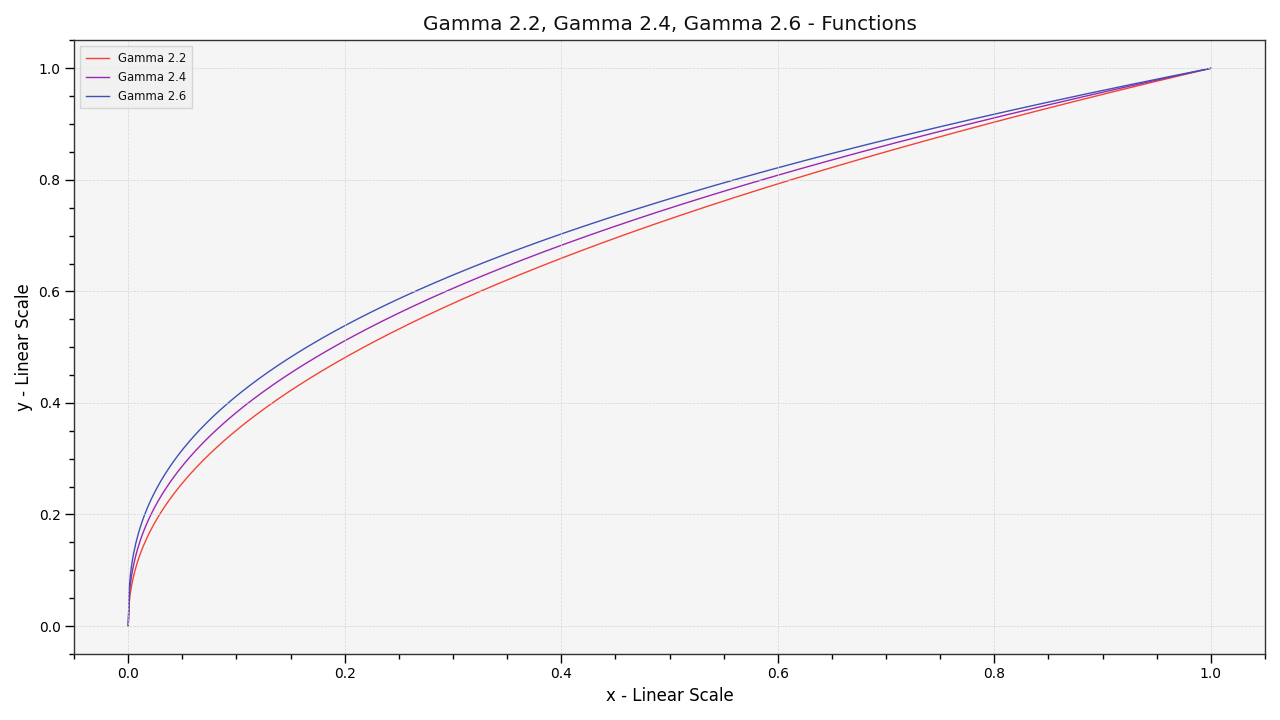# colour.plotting.plot_multi_functions#

colour.plotting.plot_multi_functions(functions: Dict[str, Callable], samples: ArrayLike | None = None, log_x: = None, log_y: = None, plot_kwargs: dict | List[dict] | None = None, **kwargs: Any) Tuple[plt.Figure, plt.Axes][source]#

Plot given functions.

Parameters:
• functions (Dict[str, Callable]) – Functions to plot.

• samples (ArrayLike | None) – Samples to evaluate the functions with.

• log_x (int | None) – Log base to use for the x axis scale, if None, the x axis scale will be linear.

• log_y (int | None) – Log base to use for the y axis scale, if None, the y axis scale will be linear.

• plot_kwargs (dict | List[dict] | None) – Keyword arguments for the `matplotlib.pyplot.plot()` definition, used to control the style of the plotted functions. `plot_kwargs` can be either a single dictionary applied to all the plotted functions with the same settings or a sequence of dictionaries with different settings for each plotted function.

• kwargs (Any) – {`colour.plotting.artist()`, `colour.plotting.render()`}, See the documentation of the previously listed definitions.

Returns:

Current figure and axes.

Return type:

`tuple`

Examples

```>>> functions = {
...     "Gamma 2.2": lambda x: x ** (1 / 2.2),
...     "Gamma 2.4": lambda x: x ** (1 / 2.4),
...     "Gamma 2.6": lambda x: x ** (1 / 2.6),
... }
>>> plot_multi_functions(functions)
...
(<Figure size ... with 1 Axes>, <...Axes...>)
```